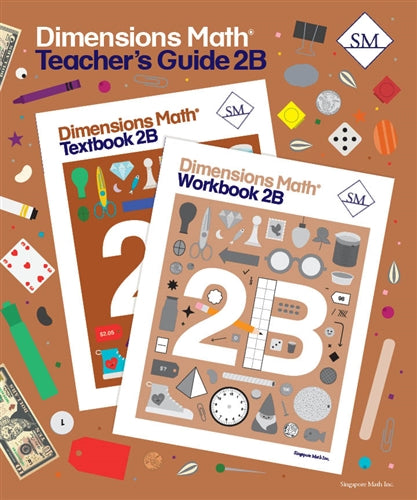# Dimensions Math Teacher's Guide 2B

Dimensions Math® Teacher's Guide 2B

Chapter 8: Mental Calculation

Lesson 3: Making 100
Lesson 4: Adding 97, 98, or 99
Lesson 5: Practice A
Lesson 6: Subtracting Ones Mentally
Lesson 7: Subtracting Tens Mentally
Lesson 8: Subtracting 97, 98, or 99
Lesson 9: Practice B
Lesson 10: Practice C

Chapter 9: Multiplication and Division of 3 and 4

Lesson 1: The Multiplication Table of 3
Lesson 2: Multiplication Facts of 3
Lesson 3: Dividing by 3
Lesson 4: Practice A
Lesson 5: The Multiplication Table of 4
Lesson 6: Multiplication Facts of 4
Lesson 7: Dividing by 4
Lesson 8: Practice B
Lesson 9: Practice C

Chapter 10: Money

Lesson 1: Making \$1
Lesson 2: Dollars and Cents
Lesson 3: Making Change
Lesson 4: Comparing Money
Lesson 5: Practice A
Lesson 7: Subtracting Money
Lesson 8: Practice B

Chapter 11: Fractions

Lesson 1: Halves and Fourths
Lesson 2: Writing Unit Fractions
Lesson 3: Writing Fractions
Lesson 4: Fractions that Make 1 Whole
Lesson 5: Comparing and Ordering Fractions
Lesson 6: Practice
Review 3

Chapter 12: Time

Lesson 1: Telling Time
Lesson 2: Time Intervals
Lesson 3: A.M. and P.M.
Lesson 4: Practice

Chapter 13: Capacity

Lesson 1: Comparing Capacity
Lesson 2: Units of Capacity
Lesson 3: Practice

Chapter 14: Graphs

Lesson 1: Picture Graphs
Lesson 2: Bar Graphs
Lesson 3: Practice

Chapter 15: Shapes

Lesson 1: Straight and Curved Sides
Lesson 2: Polygons
Lesson 3: Semicircles and Quarter-circles
Lesson 4: Patterns
Lesson 5: Solid Shapes
Lesson 6: Practice
Review 4
Review 5

Dimensions Math® PreK-5 series features the progression, rigor, and pacing that define Singapore math. Throughout the series, five characters offer students suggestions on how to think about problems. They remind students of strategies they’ve learned and point out important information that encourages them to come up with their own solutions.

Textbook lessons begin with a task that allows students to apply their previous knowledge and learn through discussion. Once students have mastered a concept with the use of concrete and pictorial aids, they are ready to take on more abstract mathematical problem sets. They reach fluency by collecting various strategies along the way and applying them to new problems. Word problems give students a sense of math in real-world contexts.

Workbooks offer independent practice that follows a careful progression of exercise variation. Each textbook lesson includes a corresponding workbook exercise that starts with pictorial representation and progresses to more challenging abstract problems. Workbooks for PreK-2 are perforated.

Teacher’s Guides include lesson plans, mathematical background, games, helpful suggestions, and comprehensive resources for daily lessons. Lessons are laid out clearly and activities are designed for the whole class, small groups, and extension.

Textbooks and Workbooks do not include answer keys. Answers are in Teacher's Guides.
ISBN  9781947226074